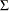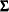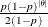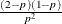### Sampling Methods

When suitable, PROC MCMC chooses the optimal sampling method for each parameter. That involves direct sampling either from the conditional posterior via conjugacy (see the section Conjugate Sampling) or via the marginal posterior (see the section Direct Sampling). Alternatively, PROC MCMC samples according to Table 59.5. Each block of parameters is classified by the nature of the prior distributions. “Continuous” means all priors of the parameters in the same block have a continuous distribution. “Discrete” means all priors are discrete. “Mixed” means that some parameters are continuous and others are discrete. Parameters that have binary priors are treated differently, as indicated in the table.

Table 59.5: Sampling Methods in PROC MCMC

Blocks

Default Method

Alternative Method

Continuous

Multivariate normal (MVN)

Multivariate t (MVT); slice sampler

Discrete (other than binary)

Binned MVN

Binned MVT or symmetric geometric

Mixed

MVN

MVT

Binary (single dimensional)

Inverse CDF

Binary (multidimensional)

Independence sampler

For a block of continuous parameters, PROC MCMC uses a multivariate normal distribution as the default proposal distribution. In the tuning phase, PROC MCMC finds an optimal scale c and a tuning covariance matrix.

For a discrete block of parameters, PROC MCMC uses a discretized multivariate normal distribution as the default proposal distribution. The scale c and covariance matrixare tuned. Alternatively, you can use an independent symmetric geometric proposal distribution. The density has formand has variance. In the tuning phase, the procedure finds an optimal proposal probability p for every parameter in the block.

You can change the proposal distribution, from the normal to a t distribution. You can either use the PROC option PROPDIST=T(df) or PARMS statement option </ T(df)> to make the change. The t distributions have thicker tails, and they can propose to the tail areas more efficiently than the normal distribution. It can help with the mixing of the Markov chain if some of the parameters have a skewed tails. See Nonlinear Poisson Regression Models. The independence sampler (see the section Independence Sampler in Chapter 7: Introduction to Bayesian Analysis Procedures,) is used for a block of binary parameters. The inverse CDF method is used for a block that consists of a single binary parameter.

For parameters with continuous prior distributions, you can use the slice sampler as an alternative sampling algorithm. To do so, specify the SLICE option in the PARMS. When you specify the SLICE option, all parameters are updated individually. PROC MCMC does not support a multivariate version of the slice sampler. For more in information about the slice sampler, see the sectionSlice Sampler in Chapter 7: Introduction to Bayesian Analysis Procedures.

The sampling algorithms for the random-effects parameters are chosen in a similar fashion. The preferred algorithms are the direct method either from the full conditional or the marginal. When these are not attainable, Metropolis with normal proposal becomes the default for continuous random-effects parameters, and discrete Metropolis with normal proposal becomes the default for discrete random-effects parameters. You can use the ALGORITHM= option in the RANDOM statement to choose the slice sampler or discrete Metropolis with symmetric geometric as the alternatives.

The sampling preference of the missing data variables is the same as the random-effects parameters. The reserve sampling algorithm is the Metropolis. There is no alternative sampling method available for the missing data variables.